Kitchen

Kitchen roller has a diameter 70 mm and width of 359 mm. How many square millimeters roll on one turn?

Result

S =  78948.2 mm2

Solution:Leave us a comment of example and its solution (i.e. if it is still somewhat unclear...):Be the first to comment!Next similar examples:

1. Roller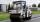Roller has a diameter of 0.96 m and a width 169 cm. How many m2 of road level when he turns 42-times?
2. GreenhouseGarden plastic greenhouse is shaped half cylinder with a diameter of 6 m and base length 20 m. At least how many m2 of plastic is need to its cover?
3. Reservoir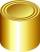6 m long reservoir has a diameter of 2.2 m. What is its surface area in square meters?
4. PipeHow long is the pipe with an outside diameter of 1.44 m if his coloring consumed 44 kg of color. 1 kg of color coverage is 9 m2.
5. Cylinder diameter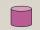The surface of the cylinder is 149 cm2. The cylinder height is 6 cm. What is the diameter of this cylinder?
6. Concrete pipe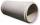Concrete pipe is cylindrical with an inner diameter 110 cm and outer 120 cm. Calculate the surface of the concrete pipe, if it is 9 m long.
7. Fit ballWhat is the size of the surface of Gymball (FIT - ball) with a diameter of 65 cm?
8. Rotary cylinder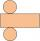The rotating cylinder has a surface area 69.08 cm2. The area of the shell is 62.8 cm 2. What is the diameter of the cylinder?
9. Highway repairThe highway repair was planned for 15 days. However, it was reduced by 30%. How many days did the repair of the highway last?
10. PercentsHow many percents is 900 greater than the number 750?
11. Cylinder surface areaVolume of a cylinder whose height is equal to the radius of the base is 678.5 dm3. Calculate its surface area.
12. CylinderThe cylinder surface is 859 dm2, its height is equal to the radius of the base. Calculate height of this cylinder.
13. Concrete pipe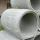How much will cost cover a 6 m long concrete pipe with an outer radius 1.5 m and inner radius 0.8 meters if 1 m2 paint costs 24 €.
14. Linear systemSolve this linear system (two linear equations with two unknowns): x+y =36 19x+22y=720
15. Cylinder - A&VThe cylinder has a volume 1287. The base has a radius 10. What is the area of surface of the cylinder?
16. Center traverseIt is true that the middle traverse bisects the triangle?
17. Cylinder - basicsCylinder with base radius r = 45 dm and height h=22 dm. Calculate: